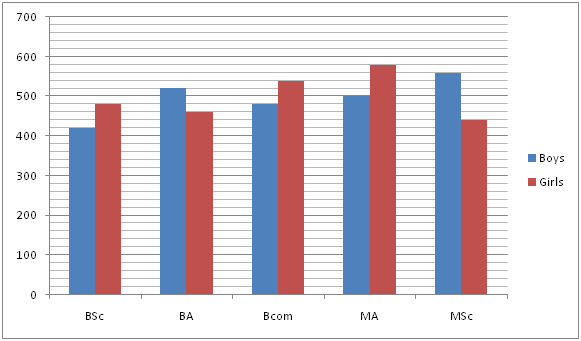# IBPS PO Prelims Quantitative Aptitude Questions 2019 (Day-5)

Dear Aspirants, Our IBPS Guide team is providing new series of Quantitative Aptitude Questions for IBPS PO Prelims 2019 so the aspirants can practice it on a daily basis. These questions are framed by our skilled experts after understanding your needs thoroughly. Aspirants can practice these new series questions daily to familiarize with the exact exam pattern and make your preparation effective.

Check here for IBPS PO Prelims Mock Test 2019

##### Check here for IBPS PO  Mock Test 2019
[WpProQuiz 6945]

Directions (1 – 5): What value should come in the place of (?) in the following questions?

1) 75 % of 320 – 24 % of 150 + 80 % of 280 = ?

a) 428

b) 432

c) 436

d) 424

e) 421

2) 2030 ÷ √841 + 12.5 * 24 + ? = 47 * 11

a) 143

b) 147

c) 151

d) 156

e) 161

3) 6300 ÷ 35 + 15 * 28 – 60 % of 420 = ?

a) 342

b) 345

c) 348

d) 352

e) 358

4) 212 + ?2 – 32= 45 % of 180 + 80 * 8

a) 16

b) 17

c) 18

d) 19

e) 20

5) 315 ÷ √225 – 6.5 * 12 + 55% of 240 = ?

a) 65

b) 75

c) 85

d) 55

e) 115

Directions (6 – 10): Study the following information carefully and answer the questions given below.

The given line graph shows the number of boys and girls studied in five different courses – BSc, BA, Bcom, MA and MSc in 2018.6) The number of girls studied in Bcom and MSc together is approximately what percent of the number of boys studied in BSc and MA together?

a) 106.5%

b) 108.5%

c) 110.5%

d) 104.5%

e) 102.5%

7) What is the average number of boys from all the courses together?

a) 492

b) 496

c) 500

d) 498

e) 504

8) What is the ratio of the number of girls studied in Bcom to MSc?

a) 27: 22

b) 23: 18

c) 28: 23

d) 21: 11

e) None of these

9) The number of boys studied in Bcom is approximately what percent of the total number of students studied in Bcom?

a) 43%

b) 45%

c) 47%

d) 49%

e) 51%

10) What is the difference between the total number of students studied in BSc and the total number of students studied in BA?

a) 80

b) 100

c) 90

d) 120

e) 30

Direction (1-5) :

75 % of 320 – 24 % of 150 + 80 % of 280 = ?

240 – 36 + 224 = ?

? = 428

2030 ÷ √841 + 12.5 * 24 + ? = 47 * 11

70 + 300 + ? = 517

? = 147

6300 ÷ 35 + 15 * 28 – 60 % of 420 = ?

180 + 420 – 252 = ?

348 = ?

212 + ?2 – 32= 45 % of 180 + 80 * 8

441 + ?2 – 9 = 81 + 640

?2 = 289

? = 17

315 ÷ √225 – 6.5 * 12 + 55% of 240 = ?

21 – 78 + 132 = ?

? = 75

Direction (6-10) :

Required percentage = [(540 + 440)/(420 + 500)] * 100

= 106.52%

Average number of boys = (420 + 520 + 480 + 500 + 560) / 5

= 496

Required ratio = 540: 440

= 27: 22

Required percentage = [480/(480 + 540)] * 100

= 47%